Ex 4.3

Chapter 4 Class 10 Quadratic Equations
Serial order wise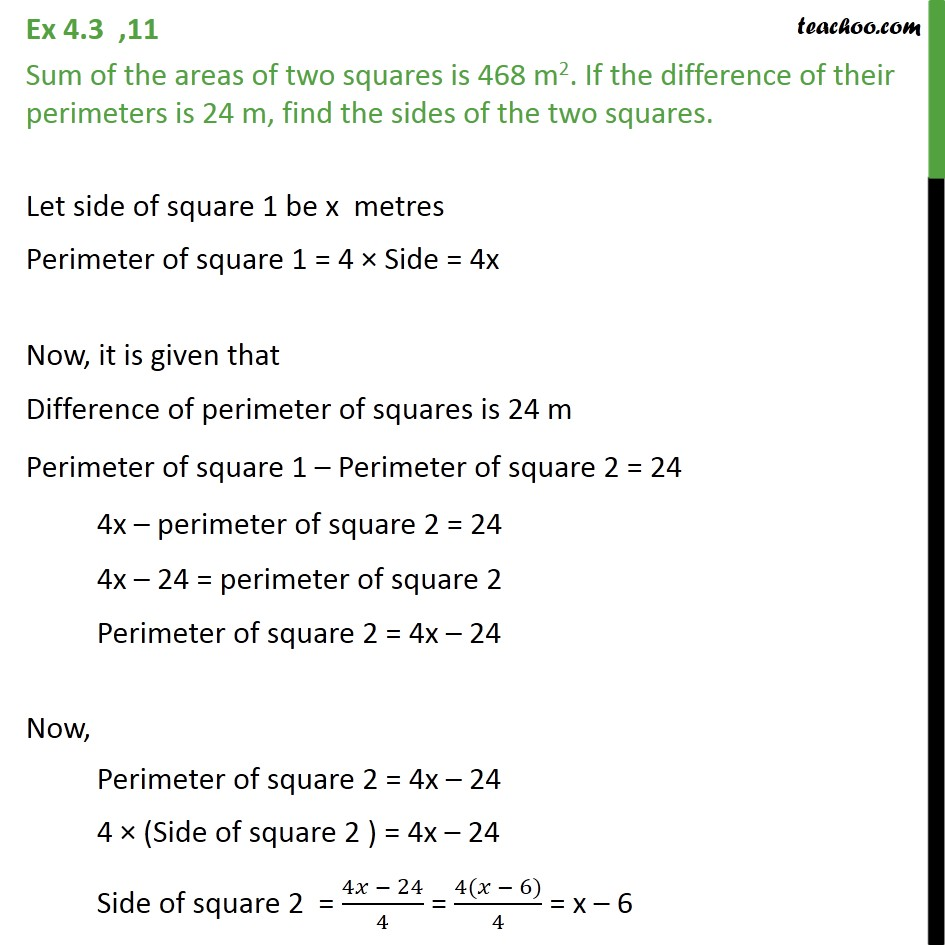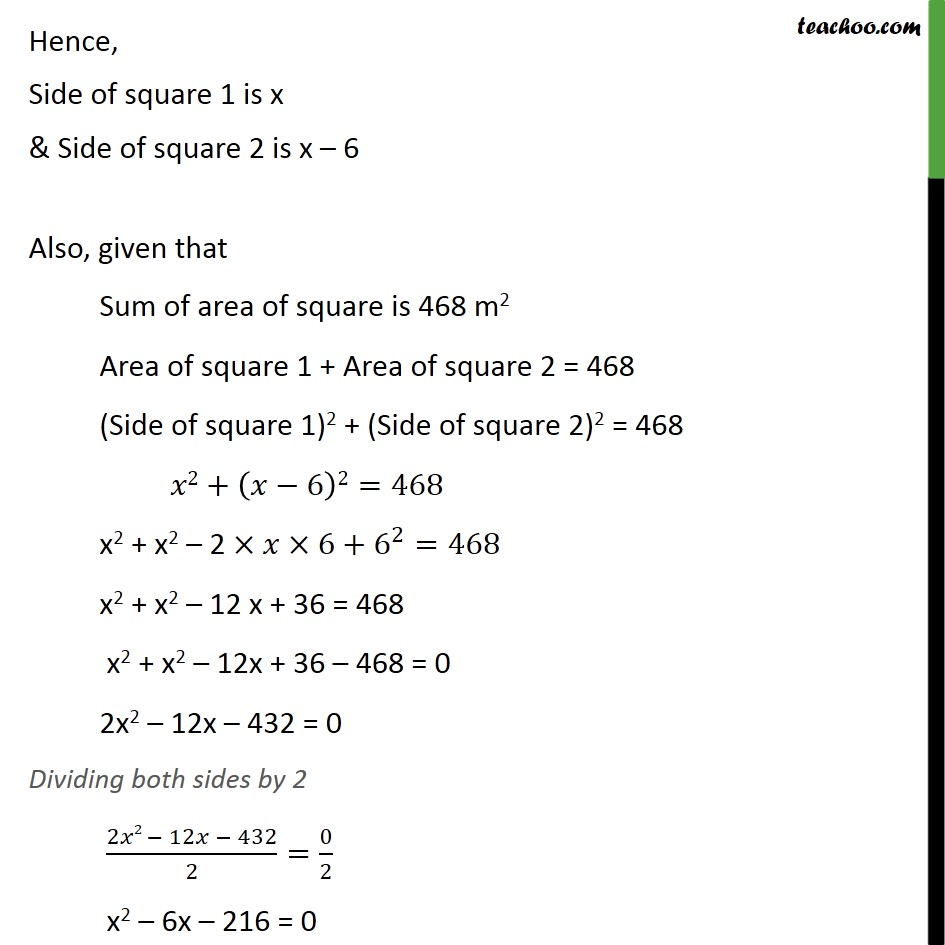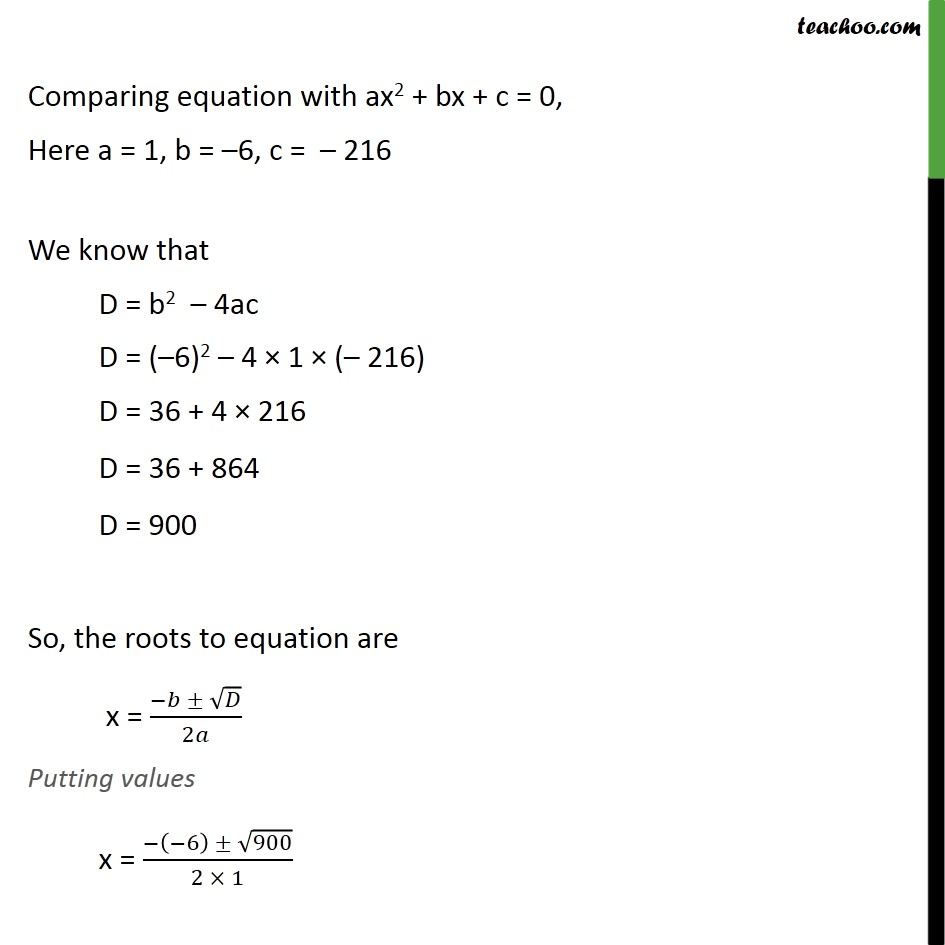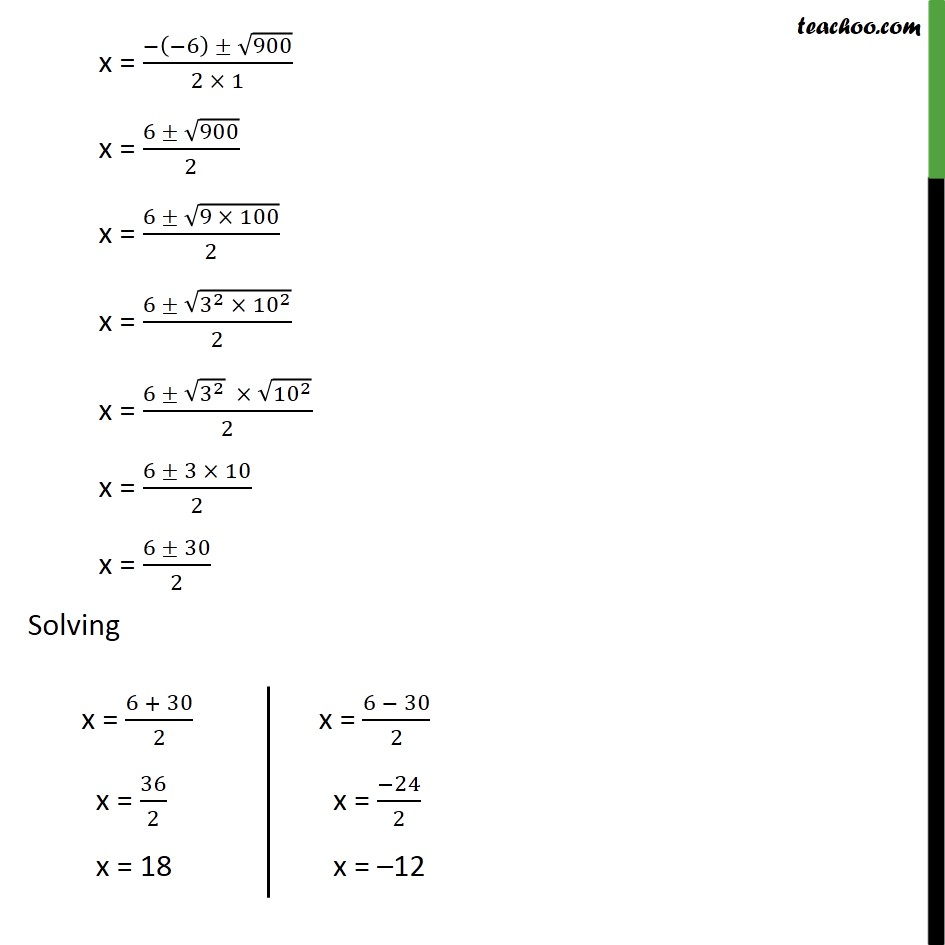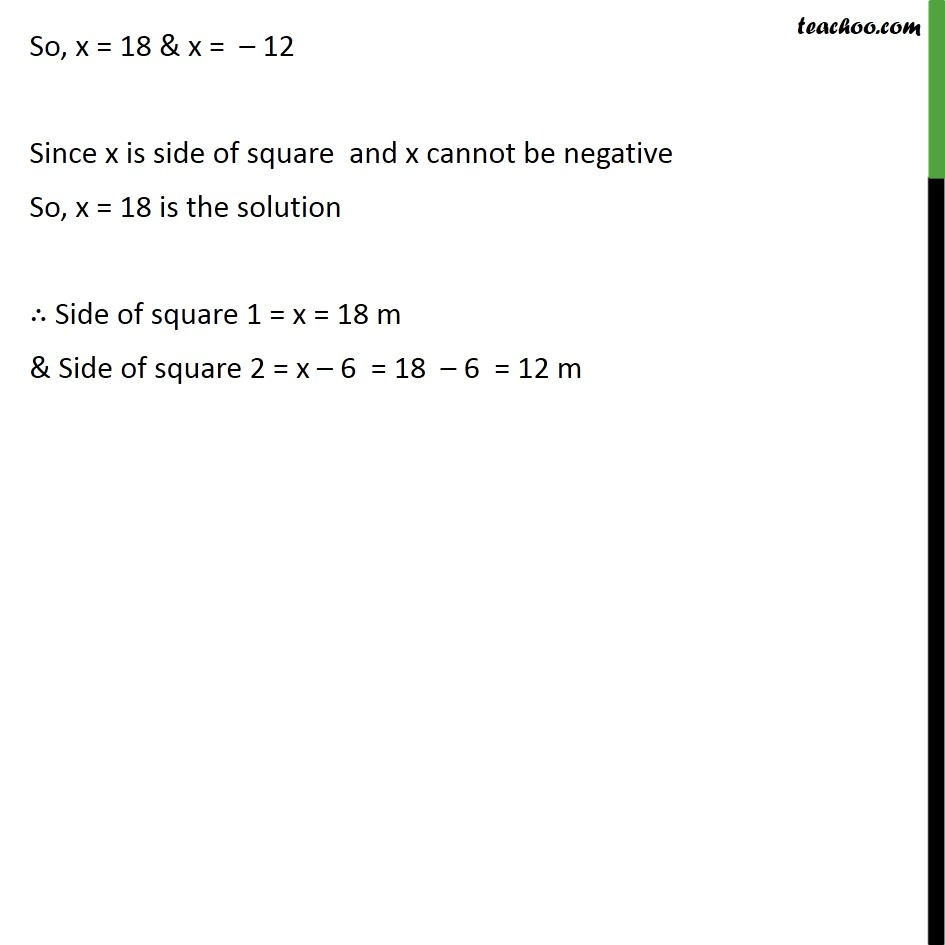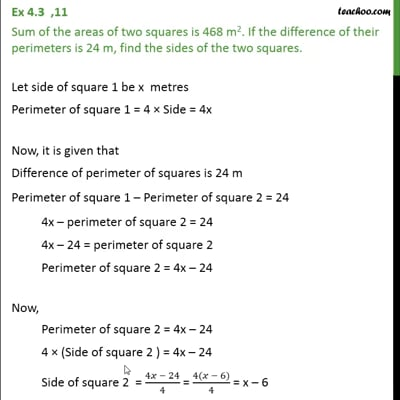This video is only available for Teachoo black users

Solve all your doubts with Teachoo Black (new monthly pack available now!)

### Transcript

Ex 4.3 ,11 Sum of the areas of two squares is 468 m2. If the difference of their perimeters is 24 m, find the sides of the two squares. Let side of square 1 be x metres Perimeter of square 1 = 4 × Side = 4x Now, it is given that Difference of perimeter of squares is 24 m Perimeter of square 1 – Perimeter of square 2 = 24 4x – perimeter of square 2 = 24 4x – 24 = perimeter of square 2 Perimeter of square 2 = 4x – 24 Now, Perimeter of square 2 = 4x – 24 4 × (Side of square 2 ) = 4x – 24 Side of square 2 = (4𝑥 − 24)/4 = (4(𝑥 − 6))/4 = x – 6 Hence, Side of square 1 is x & Side of square 2 is x – 6 Also, given that Sum of area of square is 468 m2 Area of square 1 + Area of square 2 = 468 (Side of square 1)2 + (Side of square 2)2 = 468 𝑥2+(𝑥−6)2=468 x2 + x2 – 2 ×𝑥×6+6^2=468 x2 + x2 – 12 x + 36 = 468 x2 + x2 – 12x + 36 – 468 = 0 2x2 – 12x – 432 = 0 Dividing both sides by 2 (2𝑥2 − 12𝑥 − 432)/2=0/2 x2 – 6x – 216 = 0 Comparing equation with ax2 + bx + c = 0, Here a = 1, b = –6, c = – 216 We know that D = b2 – 4ac D = (–6)2 – 4 × 1 × (– 216) D = 36 + 4 × 216 D = 36 + 864 D = 900 So, the roots to equation are x = (−𝑏 ± √𝐷)/2𝑎 Putting values x = (−(−6) ± √900)/(2 × 1) x = (−(−6) ± √900)/(2 × 1) x = (6 ± √900)/2 x = (6 ± √(9 × 100))/2 x = (6 ± √(3^2 × 〖10〗^2 ))/2 x = (6 ± √(3^2 ) × √(〖10〗^2 ))/2 x = (6 ± 3 × 10)/2 x = (6 ± 30)/2 Solving So, x = 18 & x = – 12 Since x is side of square and x cannot be negative So, x = 18 is the solution ∴ Side of square 1 = x = 18 m & Side of square 2 = x – 6 = 18 – 6 = 12 m